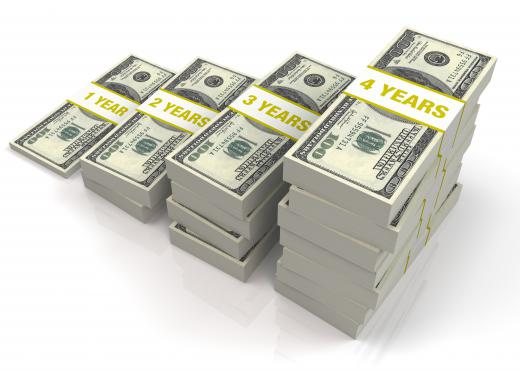Finance
Fact Checked

# In Finance, what is a Real Return?

Alexis W.
Alexis W.

The real return, also called the real rate of return, refers to the amount of money an investor actually makes on his investment. It is the amount an investment pays, after fees, commissions and dividends are adjusted for. By evaluating the real return, an investor can determine how much he is actually making on an investment to determine if the investment is a good deal or a bad deal.

Many investments are presented in terms of nominal rate of return. Nominal rate of return is the stated percentage that an investor will make. For example, if an investor opens a bank account or buys a certificate of deposit (CD), he is told the interest rate that investment will pay. The bank may say that the account pays five percent interest.

This quoted interest figure is the nominal rate of return. It does not account for inflation. It is simply the amount of total interest paid on the investment.

If inflation is three percent, however, the actual amount of interest an investor earns is less than five percent. Three percent of that interest cost is simply covered by inflation. The real rate of return on the investment, therefore, is actually only two percent.

Understanding the real return is essential to understanding how well an investment performs and to understanding how much cash the investment will generate for an investor. If an investor wants to retire, for example, he will primarily be concerned with how much his investments will give him to live on. Thus he is concerned with the amount of purchasing power his interest dollars will buy.Certificate of deposit accounts -- commonly called CDs -- require letting a bank hold a certain amount of money over a period of months or years, and the payoff is high interest rates.

In order to determine the purchasing power he will have if he tries to live off the interest of his investments, he must know the real return. Assuming that he will make five percent a year on an investment with a nominal return of five percent a year would be a grave error. Because the purchasing power of a dollar would fall three percent with a three percent inflation rate, he would actually only be living on two percent instead of on the five percent that he expected.

Calculating the real return is simple. Determine any external factors that cause the value of a saved dollar to rise. Then, subtract those external factors from the nominal rate of return to find out what the real rate of return will be.

## You might also Like